# ML Wiki

## Dot Plots

This is a Plot that is used to show only one variable

### Dot Plot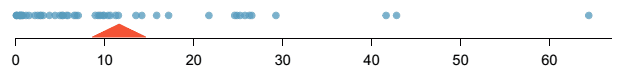It also shows

• the mean of the distribution (as the "balanced point" of this distribution)

### Stacked Dot Plot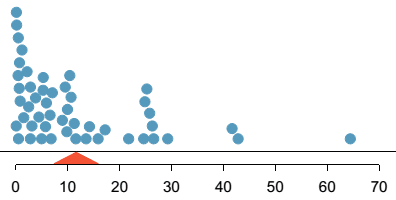• The same, but the dots are stacked on top of each other
• As the number of values grows, it becomes harder to read
• Note that this gives us the same information as Histograms - but in there, the values are binned

## R

In R, function stripchart draws the dot plot

Example with email50 data from OpenIntro:

library(openintro)
data(email50)


stripchart(email50$num_char, pch=19, col=rgb(0, 0, 1, 0.3), cex=1.5, axes=F, ylim=c(0.9, 1.5)) axis(side = 1) m = mean(email50$num_char)
polygon(x=c(m-3, m, m+3), y=c(0.90, 0.95, 0.90), col="red")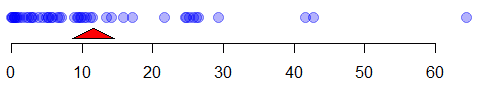Also we can add some jitter to have an idea of how many items we have in some area

set.seed(10)
stripchart(email50$num_char, method="jitter", pch=19, col=rgb(0, 0, 1, 0.3), cex=1.5, axes=F, ylim=c(0.75, 1.6)) axis(side = 1) polygon(x=c(m-3, m, m+3), y=c(0.87, 0.95, 0.87) - 0.1, col="red")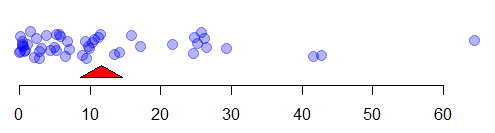Or can plot it vertically stripchart(email50$num_char, method="jitter",
vertical=T,
pch=19, col=rgb(0, 0, 1, 0.3), cex=1.5)


To have a stacked plot, use method="stack"

stripchart(round(email50$num_char), method="stack", pch=19, col=rgb(0, 0, 1, 0.5), axes=F, ylim=c(0.8, 1.8)) axis(side = 1) polygon(x=c(m-3, m, m+3), y=c(0.87, 0.95, 0.87), col="red")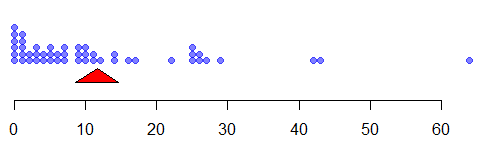Note that it given similar information to a Histogram • but the latter is binned, and this is not (so it looks rather as a Bar Chart) t = table(round(email50$num_char))
a = rep(NA, 65)
names(a) = 0:64

for (i in names(a)) {
a[i] = t[i]
}

a[is.na(a)] = 0
barplot(a, ylim=c(-0.4, max(a)))
polygon(x=c(m-3, m, m+3), y=c(-0.4, -0.05, -0.4), col="red")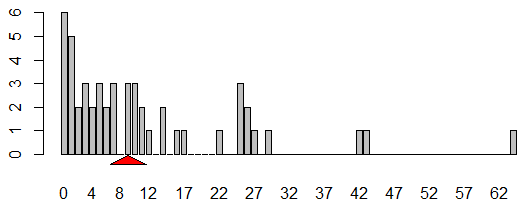hist(email50\$num_char, breaks=30, col="red")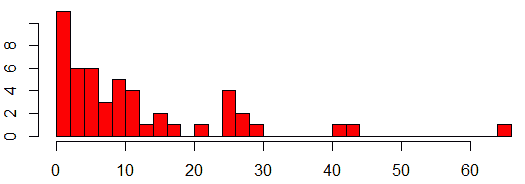And finally, an example from 

set.seed(1)
A = sample(0:10, 100, replace=T)
stripchart(A, method="stack", offset=.5, at=.15, pch = 19,
main = "Dotplot of Random Values", xlab = "Random Values")